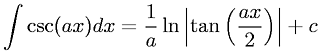Equations > Calculus > Integration/Integrals > Integral of cosecant

### Integral of cosecantLatex Code:

MathML Code:

 $\int \mathrm{csc}\left(\mathrm{ax}\right)\mathrm{dx}=\frac1a\mathrm{ln}|\mathrm{tan}\left(\frac\mathrm{ax}2\right)|+c$

MathType 5.0: